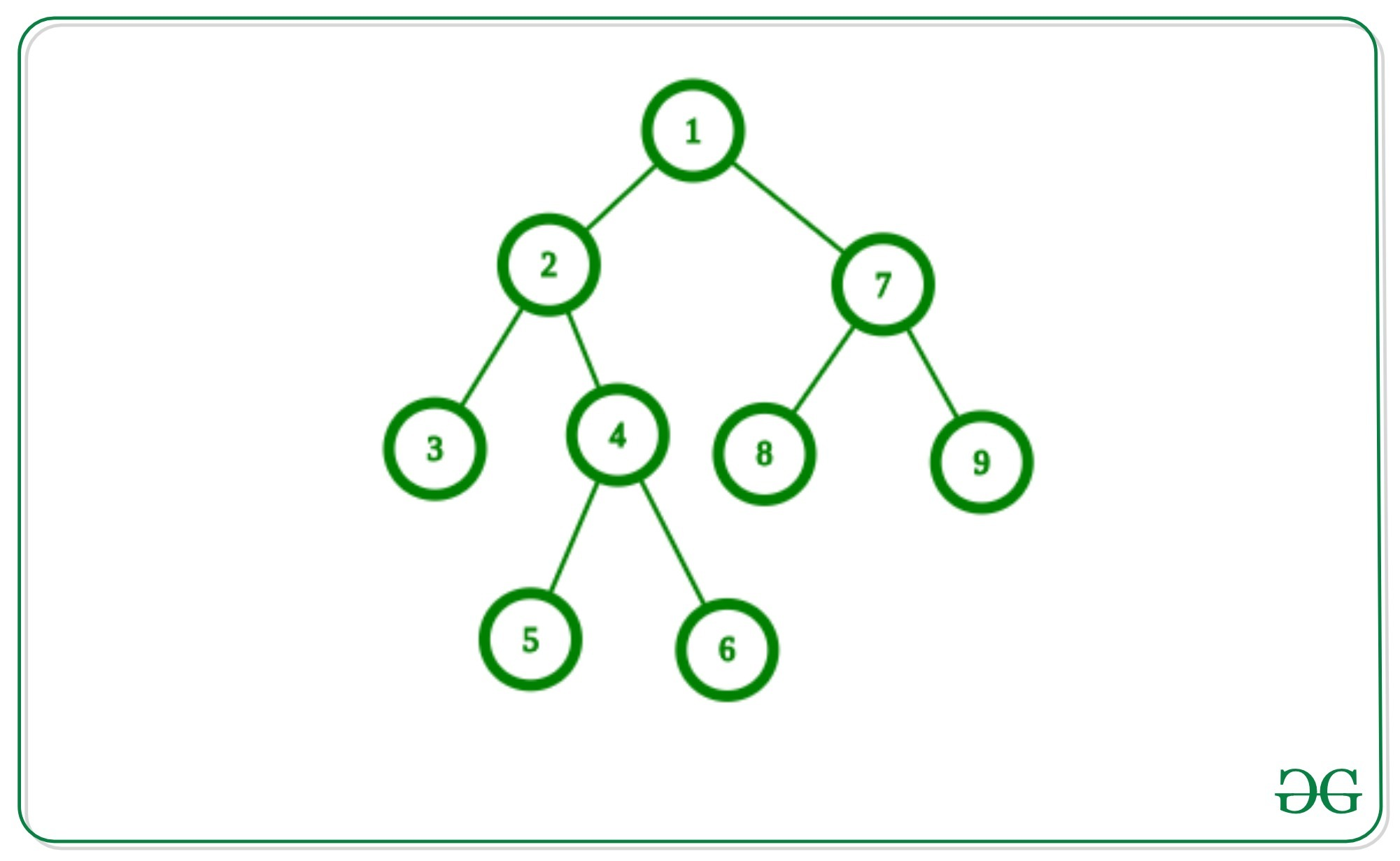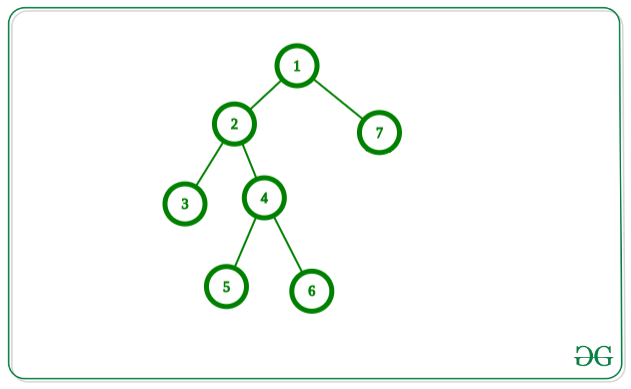# Find maximum matching in a given Binary Tree

• Last Updated : 23 Feb, 2022

Given a Tree with N nodes values from 1 to N and N – 1 edges. The task is to find the maximum matching in the given tree.

A matching in a tree is a collection of edges such that no pair of edges share a common node. Matching with the most edges is known as a maximum matching.

Examples:

Input: Below is the given graph:Output:
Explanation:
Set of Edges in the above graph for maximum matching:
(4, 5), (1, 2), (7, 8)

Input: Below is the given graph:Output:
Explanation:
Set of Edges in the above graph for maximum matching:
(4, 5), (2, 3), (1, 7)

Approach: This problem can be solved using Greedy Approach and the idea is to use post-order traversal in the tree and start with leaf edges and go up the order. Below are the steps:

1. Perform DFS Traversal on the given tree with rooted node 1 and make parent as 0 and pass current nodes as a parent of the node in recursive DFS traversal.
2. While performing traversal, for each node U and its parent node P if these nodes are unvisited then mark these nodes as visited and increment the maximum matching count by 1.
3. Print the count of a maximum matching in the above step after DFS Traversal.

The Greedy algorithm is to repeatedly take any leaf-edge.

```TreeMatch(F:forest)
M <- []
while F nonempty do {
select any leaf-edge e
M <- M + [e]
F <- F - both ends of e
}```

Why the greedy algorithm works correctly?
Let’s, assume E is a leaf edge and consider any maximum matching N. Suppose N does not contain E. Then if we add E to N, only one vertex now has two edges incident with it. So we can delete one of the edges of N and attain a maximum matching containing E.

Below is the implementation of the above approach:

## C++

 `// C++ program for the above approach``#include ``using` `namespace` `std;``#define N 10000` `// Adjacency list to store edges``vector<``int``> adj[N];` `int` `used[N];``int` `max_matching;` `// Add an edge between U and V in tree``void` `AddEdge(``int` `u, ``int` `v)``{``    ``// Edge from u to v``    ``adj[u].push_back(v);` `    ``// Edge from V to U``    ``adj[v].push_back(u);``}` `// Function that finds the maximum``// matching of the DFS``void` `Matching_dfs(``int` `u, ``int` `p)``{``    ``for` `(``int` `i = 0;``         ``i < adj[u].size(); i++) {` `        ``// Go further as we are not``        ``// allowed to go towards``        ``// its parent``        ``if` `(adj[u][i] != p) {``            ``Matching_dfs(adj[u][i], u);``        ``}``    ``}` `    ``// If U and its parent P is``    ``// not taken then we must``    ``// take &mark them as taken``    ``if` `(!used[u] and !used[p] and p != 0) {` `        ``// Increment size of edge set``        ``max_matching++;``        ``used[u] = used[p] = 1;``    ``}``}` `// Function to find the maximum``// matching in a graph``void` `maxMatching()``{``    ``// Taking 1 as a root of the tree``    ``Matching_dfs(1, 0);` `    ``// Print maximum Matching``    ``cout << max_matching << ``"\n"``;``}` `// Driver Code``int` `main()``{``    ``int` `n = 5;` `    ``// Joining edge between``    ``// two nodes in tree``    ``AddEdge(1, 2);``    ``AddEdge(1, 3);``    ``AddEdge(3, 4);``    ``AddEdge(3, 5);` `    ``// Function Call``    ``maxMatching();``    ``return` `0;``}`

## Java

 `// Java program for the above approach``import` `java.util.*;` `class` `GFG{``    ` `static` `final` `int` `N = ``10000``;` `// Adjacency list to store edges``@SuppressWarnings``(``"unchecked"``)``static` `Vector[] adj = ``new` `Vector[N];` `static` `int` `used[] = ``new` `int``[N];``static` `int` `max_matching;` `// Add an edge between U and V in tree``static` `void` `AddEdge(``int` `u, ``int` `v)``{``    ` `    ``// Edge from u to v``    ``adj[u].add(v);` `    ``// Edge from V to U``    ``adj[v].add(u);``}` `// Function that finds the maximum``// matching of the DFS``static` `void` `Matching_dfs(``int` `u, ``int` `p)``{``    ``for``(``int` `i = ``0``; i < adj[u].size(); i++)``    ``{``        ` `        ``// Go further as we are not``        ``// allowed to go towards``        ``// its parent``        ``if` `(adj[u].get(i) != p)``        ``{``            ``Matching_dfs(adj[u].get(i), u);``        ``}``    ``}` `    ``// If U and its parent P is``    ``// not taken then we must``    ``// take &mark them as taken``    ``if` `(used[u] == ``0` `&&``        ``used[p] == ``0` `&& p != ``0``)``    ``{``        ` `        ``// Increment size of edge set``        ``max_matching++;``        ``used[u] = used[p] = ``1``;``    ``}``}` `// Function to find the maximum``// matching in a graph``static` `void` `maxMatching()``{``    ` `    ``// Taking 1 as a root of the tree``    ``Matching_dfs(``1``, ``0``);` `    ``// Print maximum Matching``    ``System.out.print(max_matching + ``"\n"``);``}` `// Driver Code``public` `static` `void` `main(String[] args)``{``    ``for``(``int` `i = ``0``; i < adj.length; i++)``        ``adj[i] = ``new` `Vector();``        ` `    ``// Joining edge between``    ``// two nodes in tree``    ``AddEdge(``1``, ``2``);``    ``AddEdge(``1``, ``3``);``    ``AddEdge(``3``, ``4``);``    ``AddEdge(``3``, ``5``);` `    ``// Function call``    ``maxMatching();``}``}` `// This code is contributed by amal kumar choubey`

## Python3

 `# Python3 program for the above approach``N ``=` `10000` `# Adjacency list to store edges``adj ``=` `{}` `used ``=` `[``0` `for` `i ``in` `range``(N)]` `max_matching ``=` `0` `# Add an edge between U and V in tree``def` `AddEdge(u, v):``    ` `    ``if` `u ``not` `in` `adj:``        ``adj[u] ``=` `[]``    ``if` `v ``not` `in` `adj:``        ``adj[v] ``=` `[]` `    ``# Edge from u to v``    ``adj[u].append(v)` `    ``# Edge from V to U``    ``adj[v].append(u)` `# Function that finds the maximum``# matching of the DFS``def` `Matching_dfs(u, p):``    ` `    ``global` `max_matching``    ` `    ``for` `i ``in` `range``(``len``(adj[u])):` `        ``# Go further as we are not``        ``# allowed to go towards``        ``# its parent``        ``if` `(adj[u][i] !``=` `p):``            ``Matching_dfs(adj[u][i], u)` `    ``# If U and its parent P is``    ``# not taken then we must``    ``# take &mark them as taken``    ``if` `(``not` `used[u] ``and` `not` `used[p] ``and` `p !``=` `0``):``        ` `        ``# Increment size of edge set``        ``max_matching ``+``=` `1``        ``used[u] ``=` `1``        ``used[p] ``=` `1` `# Function to find the maximum``# matching in a graph``def` `maxMatching():` `    ``# Taking 1 as a root of the tree``    ``Matching_dfs(``1``, ``0``)` `    ``# Print maximum Matching``    ``print``(max_matching)``    ` `# Driver Code``n ``=` `5` `# Joining edge between``# two nodes in tree``AddEdge(``1``, ``2``)``AddEdge(``1``, ``3``)``AddEdge(``3``, ``4``)``AddEdge(``3``, ``5``)` `# Function Call``maxMatching()` `# This code is contributed by avanitrachhadiya2155`

## C#

 `// C# program for the above approach``using` `System;``using` `System.Collections.Generic;` `class` `GFG{``    ` `static` `readonly` `int` `N = 10000;` `// Adjacency list to store edges``static` `List<``int``>[] adj = ``new` `List<``int``>[N];` `static` `int` `[]used = ``new` `int``[N];``static` `int` `max_matching;` `// Add an edge between U and V in tree``static` `void` `AddEdge(``int` `u, ``int` `v)``{``    ` `    ``// Edge from u to v``    ``adj[u].Add(v);` `    ``// Edge from V to U``    ``adj[v].Add(u);``}` `// Function that finds the maximum``// matching of the DFS``static` `void` `Matching_dfs(``int` `u, ``int` `p)``{``    ``for``(``int` `i = 0; i < adj[u].Count; i++)``    ``{``        ` `        ``// Go further as we are not``        ``// allowed to go towards``        ``// its parent``        ``if` `(adj[u][i] != p)``        ``{``            ``Matching_dfs(adj[u][i], u);``        ``}``    ``}` `    ``// If U and its parent P is``    ``// not taken then we must``    ``// take &mark them as taken``    ``if` `(used[u] == 0 &&``        ``used[p] == 0 && p != 0)``    ``{``        ` `        ``// Increment size of edge set``        ``max_matching++;``        ``used[u] = used[p] = 1;``    ``}``}` `// Function to find the maximum``// matching in a graph``static` `void` `maxMatching()``{``    ` `    ``// Taking 1 as a root of the tree``    ``Matching_dfs(1, 0);` `    ``// Print maximum Matching``    ``Console.Write(max_matching + ``"\n"``);``}` `// Driver Code``public` `static` `void` `Main(String[] args)``{``    ``for``(``int` `i = 0; i < adj.Length; i++)``        ``adj[i] = ``new` `List<``int``>();``        ` `    ``// Joining edge between``    ``// two nodes in tree``    ``AddEdge(1, 2);``    ``AddEdge(1, 3);``    ``AddEdge(3, 4);``    ``AddEdge(3, 5);` `    ``// Function call``    ``maxMatching();``}``}` `// This code is contributed by amal kumar choubey`

## Javascript

 ``

Output

`2`

Time Complexity: O(V + E), where V is the number of edges and E is the number of edges.
Auxiliary Space: O(V)

Bottom-up DFS approach

Another intuitive approach to solve this problem is to use DFS in a bottom-up manner and return two values at each level

Maximum matching including the current node

Maximum matching excluding the current node

We will recurse on the left and right subtrees and get these values for both of them. We can then calculate new values for the current level based on these values.

Let left_included denote maximum matching including root of left subtree and left_excluded denote the maximum matching excluding the root of left subtree. Similarly, for right_included and right_excluded.

If we include the current node in maximum matching, then we have to exclude one of either left sub tree root or right sub tree root. Including both will cause overlapping on current node which is not allowed. By excluding either left or right sub tree root we can increase the maximum matching by 1 by including one of the edges from current_node -> left sub tree root or current_node -> right sub tree root.

Thus maximum matching including current node will be given by

current_including = max(max(left_including, right_excluding) + 1, max(left_excluding, right_including) + 1)

If we exclude the current node then we can include both left and right subtree root. As matchings in left and right subtrees are independent of each other we can get maximum value by adding both matchings.

Thus maximum matching excluding current node will be given by

current_excluding = left_including + right_including

We will return both these values from the current recursion level to upper recursion levels. After the recursion completes we will receive two values, maximum matching including root node and maximum matching excluding root node.

The maximum of those two will give the maximum matching in the tree.

## Python3

 `class` `Node:``    ``def` `__init__(``self``, key):``        ``self``.left ``=` `None``        ``self``.right ``=` `None``        ``self``.val ``=` `key``        ` `def` `max_matching_helper(root):``  ``if` `not` `root:``    ``return` `(``0``, ``0``)``  ` `  ``if` `not` `root.left ``and` `not` `root.right:``    ``return` `(``0``, ``0``)``  ` `  ``left_included, left_excluded ``=` `max_matching_helper(root.left)``  ``right_included, right_excluded ``=` `max_matching_helper(root.right)``  ` `  ``# Maximum matching gincluding current node``  ``curr_included ``=` `max``(``max``(left_included, right_excluded) ``+` `1``, ``max``(left_excluded, right_included) ``+` `1``)``  ``# Maximum matching excluding current node``  ``curr_excluded ``=` `left_included ``+` `right_included``  ` `  ``return` `(curr_included, curr_excluded)``  ` `        ` `def` `max_matching(root):``  ``# Taking 1 as a root of the tree``  ``root_including, root_excluding ``=` `max_matching_helper(root)``  ` `  ``# Return maximum Matching``  ``return` `max``(root_including, root_excluding)` `# Driver code``root ``=` `Node(``1``)``root.left ``=` `Node(``2``)``root.right ``=` `Node(``7``)``root.left.left ``=` `Node(``3``)``root.left.right ``=` `Node(``4``)``root.left.right.left ``=` `Node(``5``)``root.left.right.right ``=` `Node(``6``)``root.right.left ``=` `Node(``8``)``root.right.right ``=` `Node(``9``)` `print``(max_matching(root))` `# This code is contributed by Rathijeet Bhave`

Output

`3`

Time Complexity: O(V + E), where V is the number of edges and E is the number of edges.

Auxiliary Space: O(V)

My Personal Notes arrow_drop_up